American Journal of Civil Engineering
Volume 4, Issue 4, July 2016, Pages: 191-197

Characteristics Analysis of Load Caused by Punching Pile Construction of Highway Bridge Pile Foundation

She Yanhua

School of Urban Construction, Yangtze University, Jing Zhou, China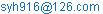She Yanhua. Characteristics Analysis of Load Caused by Punching Pile Construction of Highway Bridge Pile Foundation.American Journal of Civil Engineering. Vol. 4, No. 4, 2016, pp. 191-197. doi: 10.11648/j.ajce.20160404.19

Received: June 9, 2016; Accepted: June 21, 2016; Published: July 11, 2016

Abstract: The characteristics of impact loads caused by punching pile construction of highway bridge pile foundation and the propagation law of vibration wave in the soil were researched. Combined with site monitoring vibration signal which is generated by the bridge pile foundation construction of Zhang Shi Expressway across the Shaanxi Beijing pipeline, signal waveforms of the punching pile construction and blasting construction were analyzed contrastively. It expounded the characteristics of impact loads, and deduced the mathematical expressions of impact loads. On the basis of the analysis model, through the numerical simulation, the propagation law of vibration wave in the soil caused by the impacting point source excitation was revealed. Thus, it indicates that because of the buried pipeline, the local gradient of vibration wave velocity field increased in the area between the vibration source and the pipeline, but decreased in the far direction.

Keywords: Punching Pile Construction, Impact Loads, Vibration Wave, Propagation Law

Contents

1. Introduction

In the punching pile construction, the hammer drops down from a certain height colliding with the soil as a greater impact which is very difference from the vibration caused by general earthquake or blasting. Selecting a fragment of the typical vibration signal of construction of Zhang Jiakou to Shi Jiazhuang (Zhang-Shi) expressway across Shan xi-Beijing gas pipeline bridge pile foundation, the vibration waveform of punching pile construction is shown in Figure 1, while the Figure 2 shows the curve of maximum vibration velocity of corresponding punching depth at different measuring points. The vibration waveform of each measuring point is shown in Figure 3, while Figure 4 and Figure 5 are the typical signal spectrum analysis of the construction vibration and blasting vibration of the pile foundation separately.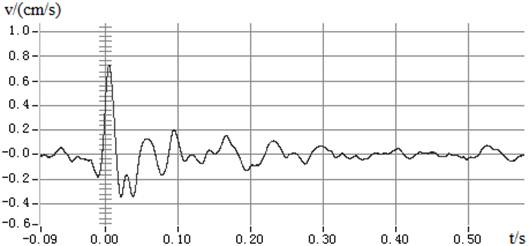(a) Distance of 5m from the source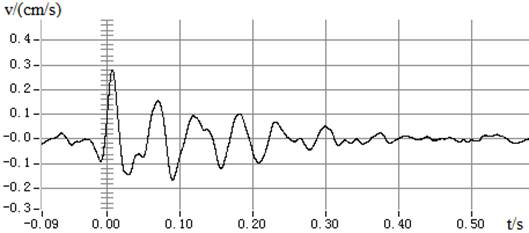(b) Distance of 7m from the source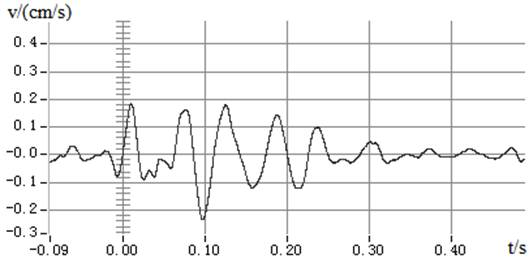(c) Distance of 10m from the source

Figure 1. Vibration vaveform in punching pile construction.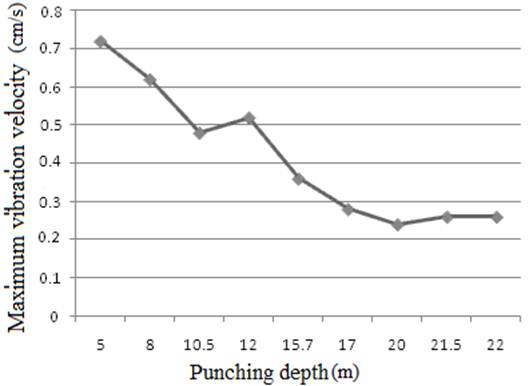Figure 2. The variation of the maximum vibration velocity of different punching depth.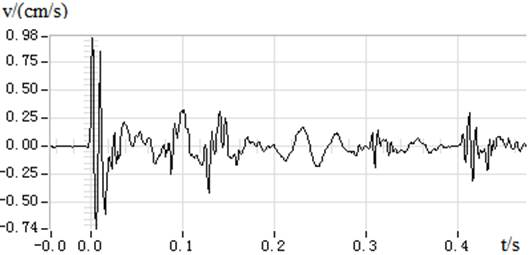(a) Distance of 5m from the source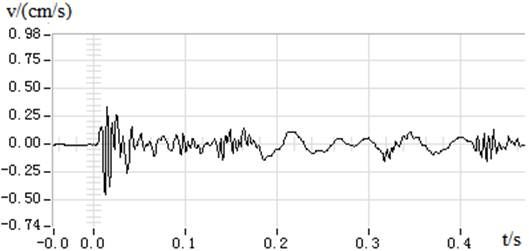(b) Distance of 7m from the source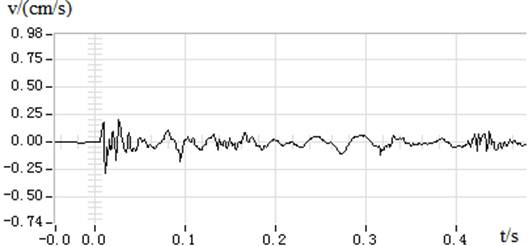(c) Distance of 10m from the source

Figure 3. Wave of blasting vibration signal.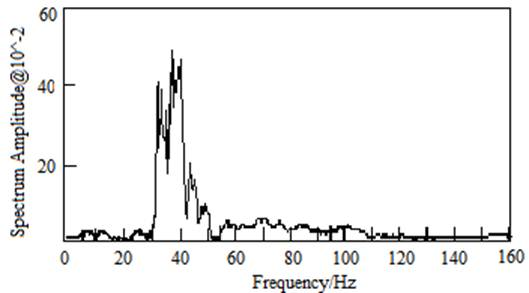Figure 4. Spectrum analysis of typical vibration signal in punching pile construction.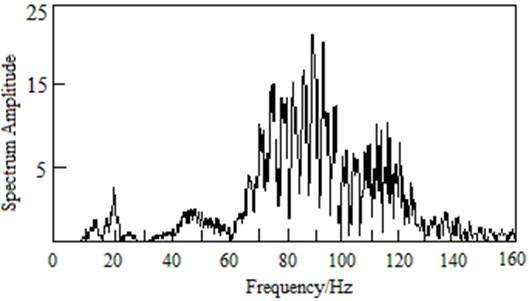Figure 5. Spectrum analysis of typical vibration signal of blasting construction.

Compared with the analysis and research of the natural and blasting vibration, the characteristics of the construction vibration of the punching pile are mainly as follows:

(1) It belongs to the instantaneous shock type point source vibration, and its action time is very short. As shown in Figure 1, despite the high amplitude of the vibration velocity, but the failure zone is limited due to rapid decay and under the action of earthquake induced the compared structure forced vibration are essentially different, and the blasting vibration effect is also of very big difference (shown in Figure 3); (2) Under normal situation, the vibration strength will decrease with pile hole depth increase. But a large number of experimental results show that the vibrations are magnified and low frequency vibration even in the presence of multiple rebound phenomenon, which may related to geological factors (shown in Figure 2); (3) Field tests illustrate that the punching pile construction vibration are generally in low-frequency region of 5-50Hz (shown in Figure 4), and the principal frequency is closer to inherent frequency of adjacent structures compared with the blasting (shown in Figure 5); (4) The serious harm on adjacent structures of the cumulative effect should not be overlooked in the follows as multiple punching, short interval, and intensive vibration period, belonging to the relatively long-term cyclic loading.

2.2. Analytical Model of Impact Load

Hammer, with a certain speed impacting on the soil layer (gravel soil or rock), due to the small relative stiffness of the soil, will rush to a certain depth of the soil after a rapid decrease to zero, and get a smaller rebound generally. This process will have a greater impact because of the very short action time. The simplified impact physical model is shown in Figure 6, and the hammer with a certain mass dropping down from the S stroke acts with the soil mass.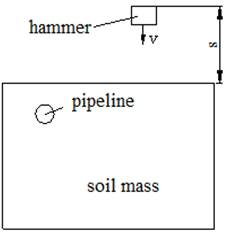Figure 6. Physical model of the hammer impact soil layer.

The hammer is regarded as a rigid body, which is assumed to be not deformed in the collision with the soil medium, not considering the gravity effect and the friction between the punch and the soil medium. The equation of motion of the hammer can be listed as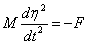(1)

In the formula (1), M—the quality of the hammer,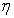— the displacement of the soil mass which is contacted with the hammer, t—the time of the hammer contacting with the soil mass, and F—the impact force generated by the hammer contacting with the soil mass.

The relationship between the effective impact force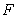and the displacementis obtained by the existing results about the contact problem of elastic theory. Approximating the contact part by paraboloid, it can obtain the following formula :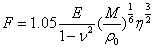(2)

In the formula (2),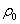— the density of the hammer,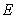— the elastic modulus of the soil medium, and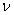— the poisson's ratio of the soil medium.

Substituting formula (2) into the formula (1), it gets the following differential equation: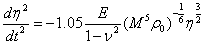(3)

Among them, the initial conditions are: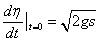(4)

The above two equations are all nonlinear differential equations, and the related solving methods provided by reference  can be obtained the maximum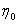and the contact time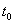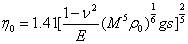(5)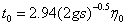(6)

Substituting the formula (5) into the formula (2), the peak impact load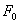can be calculated as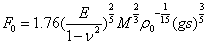(7)

As shown in Figure 7, the triangle load can be used to describe the impact load F approximately, and its expression is as follow:(8)

The dimensionless time function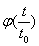is used to express the load characters and the impact load can be expressed for unity as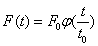(9)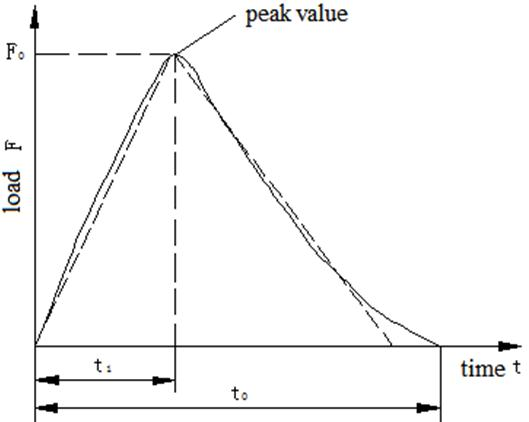3. Numerical Simulation of the Shock Wave Propagation

For the impact physical model shown in Figure 5, the ANSYS / DYNA LS- finite element analysis software is used to analyze the propagation of vibration waves generated by shock source excitation in soil mass. The 5t hammer dropping from the 3m height impacts foundation soil (sand and gravel soil). Soil model takes 100m long, 100m wide, and 15m height with homogeneous soil. In the distance 20m from the soil model center, there has a steel pipeline with buried depth of 3m. The calculation model is shown in Figure 8. The material calculation parameters are shown in Table 1.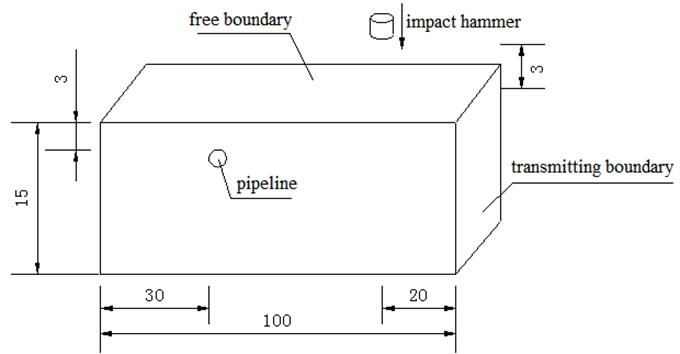Figure 8. Mechanical Calculation Model (Unit: m).

Table 1. Material Parameters and Related Calculation Conditions.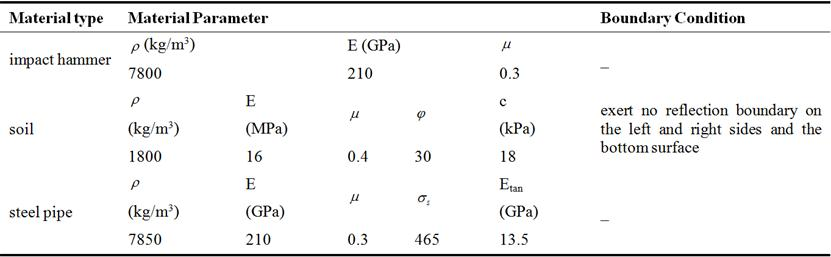According to the previous analysis, the impact of the hammer on the soil is loaded with triangular pulse load, with 0.08s duration time and the maximum load at 0.04s. The load- time curve is shown in Figure 9, and the load time value is shown in Table 2.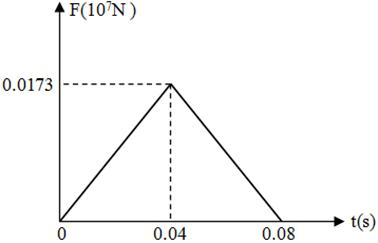Figure 9. Time History Curve of Impact Load.

Table 2. Values of Impact Load and Time Array.

 No. Time (s) Force (107N) 1 0.00 0 2 0.02 0.00865 3 0.04 0.0173 4 0.06 0.00865 5 0.08 0

The change of vibration wave propagation at different time can be expressed by animation, the numerical simulations of typical vibration wave propagation under different conditions are shown as in Figure 11 and Figure 10.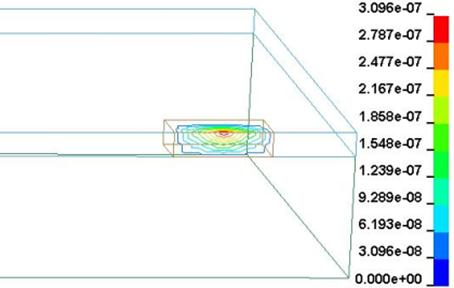(a) 0.02s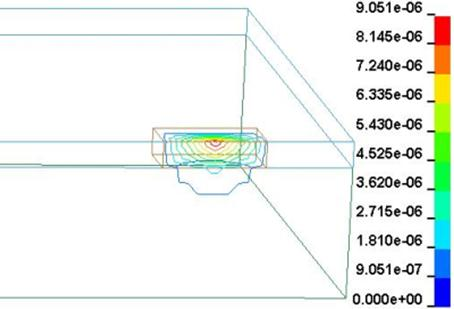(b) 0.05s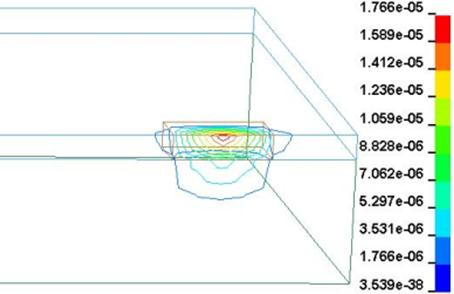(c) 0.08s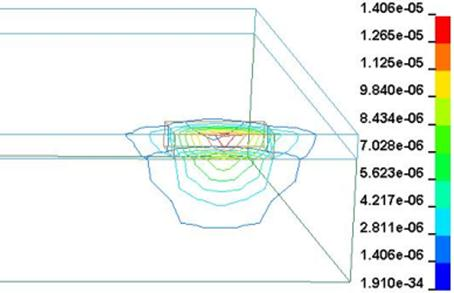(d) 0.11s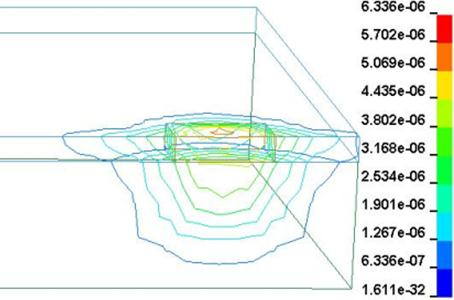(e) 0.14s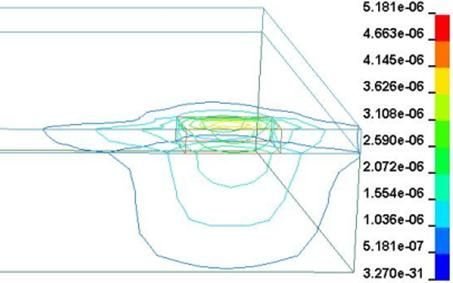(f) 0.17s

Figure 10. The Propagation of the Shock Wave in the Soil Mass.(a) 0.22s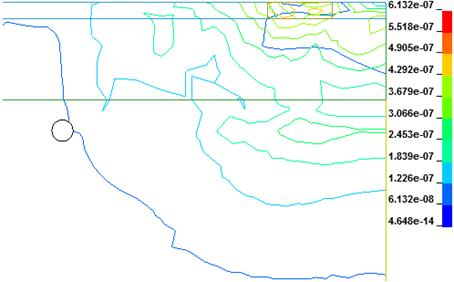(b) 0.26s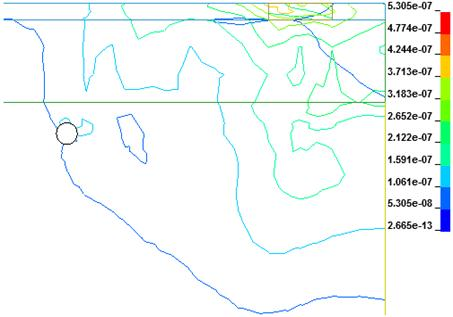(c) 0.30s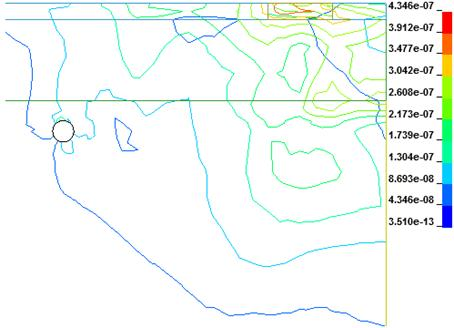(d) 0.34s

Figure 11. Influence of the Transmission of Shock Wave to the Buried Pipeline.

As shown in Figure 10, when t=0.02s, the hammer just contacting with the soil, the soil vibrates severely by shock waves. And then soil particle vibration velocity increases gradually; when t=0.08s, it reaches maximum value, and then the shock wave attenuates for the stress wave, with oval surrounding diffusion in the soil. While the peak velocity begins to decline.

It can be seen from Figure 11, when t=0.26s, the respondent stress wave is at vertical incidence to the buried pipeline attack of the lateral vibration, due to the wave front in the free surface reflecting the formation of tensile stress wave, and the toward lateral vibration velocity relative to wave front is great more than the other wave array faces; when t=0.30s, the outermost layer of wave front reaches the dorsal lateral vibration of buried pipeline, then the stress wave under pipeline diffracts where the speed is relatively slow. At the side of the pipeline, the velocity is still larger than the behind. When t=0.34s, the radius of the wave front is expanding, the back side of the pipeline is continued to spread, and its velocity is gradually fading.

The above analysis shows that the impact of vibration wave in soil mass is almost uniform when there is no buried pipeline. The existence of buried pipeline hinders the wave propagation which makes the local gradient larger in the vibrating wave velocity field and smaller in far from pipeline direction.

4. Conclusion

It has obtained the relationship between force and displacement of contact problem, and induced out the mathematical expression of the impact load, which can express by triangular load approximately; the vibration wave development process from shock source center outward propagating reveals the rules of velocity in the soil with time.

The analysis shows that existence of buried pipeline makes the vibration wave velocity field of the local gradient larger between the near field source and the pipeline, and smaller in far from pipeline field.

Acknowledgements

This work is financially supported by National Natural Science Foundation of China (NSFC, 51408057) & Youth Talent Project of Yangtze University (2015cqr06).

References

1. Zhao Xinwei, Zeng Xiangguo, Yao Anlin. et al., China Petroleum Machinery, vol. 42, 201 4, pp. 104-109.
2. Peng Xingyu, Liang Guangchuan, Zhang Peng. et al., Natural Gas Industry, vol. 32, 2012, pp. 81-84.
3. Xiong Jian, Deng Qing-lu, Zhang Hong-lianget al.,Safety and Environmental Engineering, vol. 20, 2013, pp. 108-114.
4. Xu Taolong, Zeng Xiang guo, Yao Anlin et al., Reliability Analysis of Buried Gas Pipeline Under Ramming Impact Loading Journal of Sichuan University (Engineering Science Edition), vol. 44, Supp. 2, 2012, pp. 96-100.
5. Yang Zhenkun, Du Chengwei, Building Structure, Vol. (40), 2010, pp. 328-330.
6. Li Xinliang, Li Suzhen, Shen Yong-gang, Journal of Zhejiang University (Engineering Science Edition), vol. 48, 2014, pp. 1976-1982.
7. Liu Xue tong, Zheng Shuang ying, Guo Dong dong et al., Pipeline Technique and Equipment, vol. 42, 2015, pp. 48-54.
8. Liu Jianmin, Chen Wentao, Engineering Blasting, vol. 14, 2008, pp. 20-24.
9. Fan Xisheng, Lu lai, Li Li, Journal of Vibration and Shock, vol. 15, 1996, pp. 22-26.
10. G. M. L. Gladwell, translated by Fan Tianyou. In the classical theory of elasticity contact problems [M]. Beijing: Beijing Institute of Technology press, 1991.

 Contents 1. 2. 2.1. 2.2. 3. 4.
Article ToolsAbstractPDF(2102K)